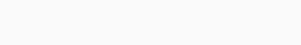Difficulty
Average 50%

Contenus

The algorithm of McNaughton and Yamada follows the next steps. Given a automaton To not states, and whose states are numbered from 1 to not, we give an expression for the languages composed of the words which label the paths of i To j, for any couple ij. This expression is built by induction using a condition on the paths. This condition states that the paths only pass through certain authorized states.

Our automaton having not states, so we focus on building a regular expression for each of not×not languages THEs, s' = { w∈X * | s.w = s'} formed by all the words that send a state s any on another state s'. If we succeed, the language recognized by the automaton will be the value of a finite union of regular expressions and therefore of a regular expression.

The idea is to proceed step by step by taking into account an increasing number of intermediate states between s and s'. The states being assumed to be numbered from 0 to not, we start with no intermediate state, then only s0, then s0 and s1, then s0s1 and s2, etc.

We notice THEs, s'(k) is the expression for the set of words which label paths from s to s' and whose intermediate vertices are less than or equal to k. The starting vertices s and ending s' are not intermediate, so they are not constrained to be less than or equal to k.

We build the THEs, s'(k) by induction on k, starting with k = 0, and ending with k = n. When k = n, the constraint on k is no longer a restriction, and THEs, s'(k)=THEs, s'  if s ≠ s', and ε +THEs, s(not)=THEs, s.

For k = 0, since the vertices are numbered starting from 1, the constraint simply expresses that there is no intermediate vertex. The only paths are transitions from s to s' (we ignore a path of length 0 in state s).

We therefore have with s = p and s' = q:

For induction, we consider a path from s to s' whose intermediate vertices are smaller than k. Two cases are then possible:

1. the intermediate vertices are smaller than k-1, then the label is in THEs, s'(k-1)
2. the path goes through state k. We then decompose the path into parts whose intermediate vertices are smaller than k-1. For this, we consider each occurrence of the vertex k in this path: between two consecutive occurrences, the intermediate vertices are smaller than k-1. We then have the formula with s = p and s' = q:.

There are therefore n + 1 steps. Each of the steps requires the computation of n² expressions, and the size of the expressions themselves increases with k.

## Example

Here is an example of McNaughton and Yamada's algorithm.

Consider the following automaton:

With k = 0 we have:

Then k = 1:

Then k = 2:

Then k = 3:

For the last step we just calculate the two expressions that interest us (initial states to final states):

The desired expression is therefore ab (a + b) ∗ + (b + aa) a ∗.FRFRENRUES